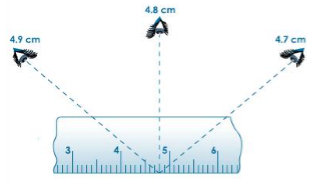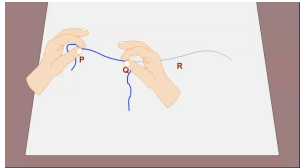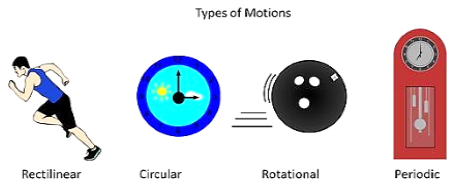# Motion and Measurement of Distances Revision Notes class 6 chapter 10

Motion and Measurement of Distances  Revision Notes class 6 chapter 10

With the changing times, transport also has gone through various modifications i.e. from animals to the invention of wheels. The evolution of transport is evident when we observe the fast cars, bullet trains etc. Even today new modes of transport are being researched upon.Fig 1: Shows the advancement in transport

• Distance: How far an object travels constitutes distance!! The GPS system introduced these days accurately measures the distance from one place to another.

• Arbitrary ways to measure the length or width:

• Measuring using handspan and measurement by a string were used in ancient times but these methods are not so reliable and hence some standard units of measurement have been introduced.Fig 2: The Handspan Method

## Standard Units of Measurement

Earlier the ‘cubit’ was accepted as a standard unit in Egypt. A cubit meant the length between fingertips and elbow. Owing to the differences in the length of body parts of each person these arbitrary systems became obsolete. Other units used earlier were ‘foot’, end of outstretched arm and chin, fist, etc.

Nowadays, the International System of units or the SI units have been accepted all over the world as a standard unit of measurement.

1 m = 100 cm

1 kg = 1000 g

1s = 1/60 min

The MKS system i.e. the metre-kilogram-second system is called the SI System.

## Correct Way to Use a Mater Scale

Step 1: Keep the scale in contact with the object to be measured.

Step 2: Start measuring from the 0 mark of the scale.

Step 3: To avoid taking incorrect measurements the eye position should be correct. Consider the following figure:The eye position in the centre is correct to get an accurate measurement, while the ones in the left and right direction will give some error in measurement.

## Measurement Along Curved Line

Is it possible to measure a curved line with a metre scale? Well, it is not so. Hence to measure a curved line the following steps can be taken into account:

• Take a thread and tie a knot at one end.

• From this end measure a small portion of the curved line which is somewhat straight and put the thumb.

• Now again start from the thumb marked position and measure another small portion of the line.

• Repeat this process until you reach the end of the line. Tie a knot on the thread on reaching the end. Now measure the two knots using a metre scale.Fig 4: Measuring A Curved Line

## Types of Motion

• Rectilinear Motion: When an object moves along a straight line, it is said to be undergoing rectilinear motion. For Example, A train moving along a railway track.

• Circular Motion: Have you seen the hands of a clock? The motion exhibited by the hands of a clock is called Circular Motion.

• Periodic Motion: When an object repeats its motion after a fixed interval of time it is said to be undergoing periodic motion. For Example, Pendulum

• Rotational Motion: This motion can be easily understood by imagining Earth’s rotation. When the Earth spins on its own axis it is said to be undergoing rotational motion.

• Motion is defined as the change in position of an object.Fig 5: Shows the examples of types of motion